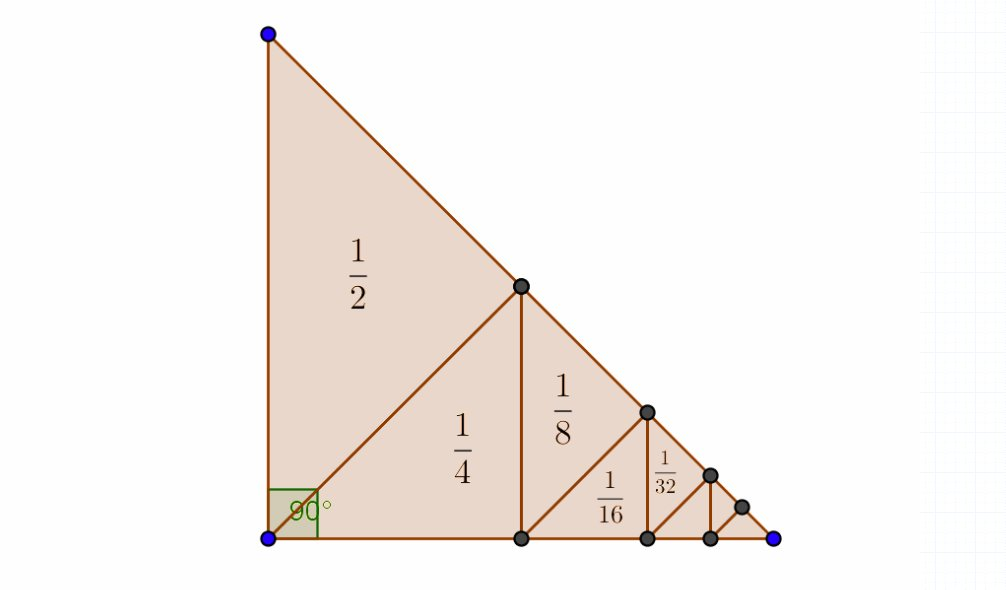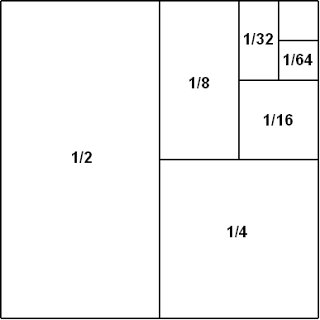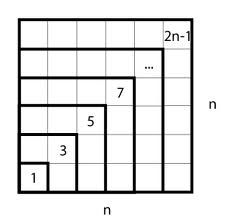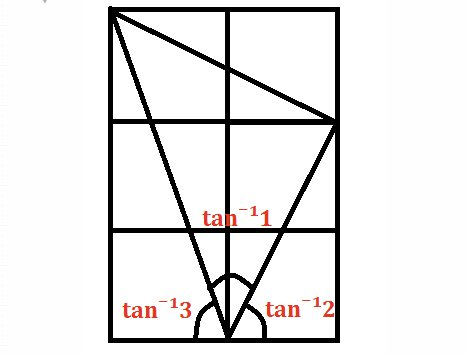## Proof Without Words #3

Proof of the identityThe figure for general n is similar, with n right pyramids, one with an (n-1)-cube of side length xk as its base and height xk for each k=1,….,n.

The derivative of sin is cosine.From ‘Proof without words‘ by Roger Nelsen

Previous ‘Proof Without Words‘: Part 1 | Part 2

M x

## Proof Without Words #2

My last post seemed to go down well so I thought I’d compile a few more images of proofs without words!Determinant is the area of a parallelogram, by Solomon Golomb, Mathematics Magazine, March 1985.A visual proof of Jensen’s inequality, found on Wikipedia. Jensen’s inequality states thatIn the diagram, the dashed curve along the X axis is the hypothetical distribution of X, while the dashed curve along the Y axis is the corresponding distribution of Y values. Note that the convex mapping Y(X) increasingly “stretches” the distribution for increasing values of X.Hope you enjoyed! M x

## Proof Without Words #1

I decided to start a new series called ‘Proof Without Words’: a collection of pictures which prove a mathematical fact with an image. Remember, these are in no way rigorous and are just meant to give an idea of why the fact is true!

### 1/2+1/4+1/8+1/16+1/32+ … = 1### Sum of the first n positive odd numbers = n^2### arctan(1) + arctan(2) + arctan(3) = π### Viviani’s Theorem

The sum of the distances from any interior point to the sides of an equilateral triangle equals the length of the triangle’s altitude.M x